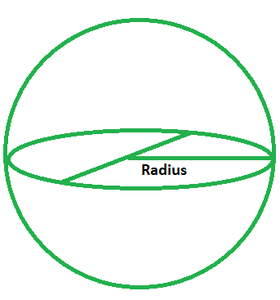# Surface Area of a Sphere Formula

• Last Updated : 31 Mar, 2022

A sphere can be called a three-dimensional equivalent of a circle. It is a collection of points in tri space that are all at the same distance from a particular point. This distance is regarded as the radius of the said sphere.### Surface Area of Sphere

The total area of a sphere refers to the region covered by the sphere’s outer surface. The three-dimensional version of a circle is known as a sphere. A sphere differs from a circle in the aspect that a circle is a two-dimensional (2D) form, but a sphere is a three-dimensional (3D) shape. A sphere’s surface area is measured in square units.

Formula

A = 4πr2

where r denotes the radius of the given sphere.

### Sample Problems

Problem 1. Find the surface area of a sphere whose radius is 5 m.

Solution:

Given: r = 5 m

Since, A = 4πr2

= 4 × 3.14 × 52

= 4 × 3.14 × 25

A = 314 m2

Problem 2. Find the surface area of a sphere of radius 10 m.

Solution:

Given: r = 10 m

Since, A = 4πr2

= 4 × 3.14 × 102

= 4 × 3.14 × 100

A = 1256 m2

Problem 3. What would be the cost of painting a sphere of radius 4.5 cm at INR 5 per sq. cm?

Solution:

Given: r = 4.5 cm

TSA = 4πr2

= 4 × 3.14 × 4.52

= 254.47 cm2

So, total cost of painting the sphere = 254.47 × 5

= INR 1272.35

Problem 4. What would be the cost of painting a sphere of radius 3 cm at INR 12 per sq. cm?

Solution:

Given: r = 4.5 cm

TSA = 4πr2

= 4 × 3.14 × 32

= 113.04 cm2

So, total cost of painting the sphere = 113.04 × 12

= INR 1356.48

Problem 5. Find the surface area of a sphere of radius 15 m.

Solution:

Given: r = 15 m

Since, A = 4πr2

= 4 × 3.14 × 152

= 4 × 3.14 × 225

A = 2826 m2

My Personal Notes arrow_drop_up